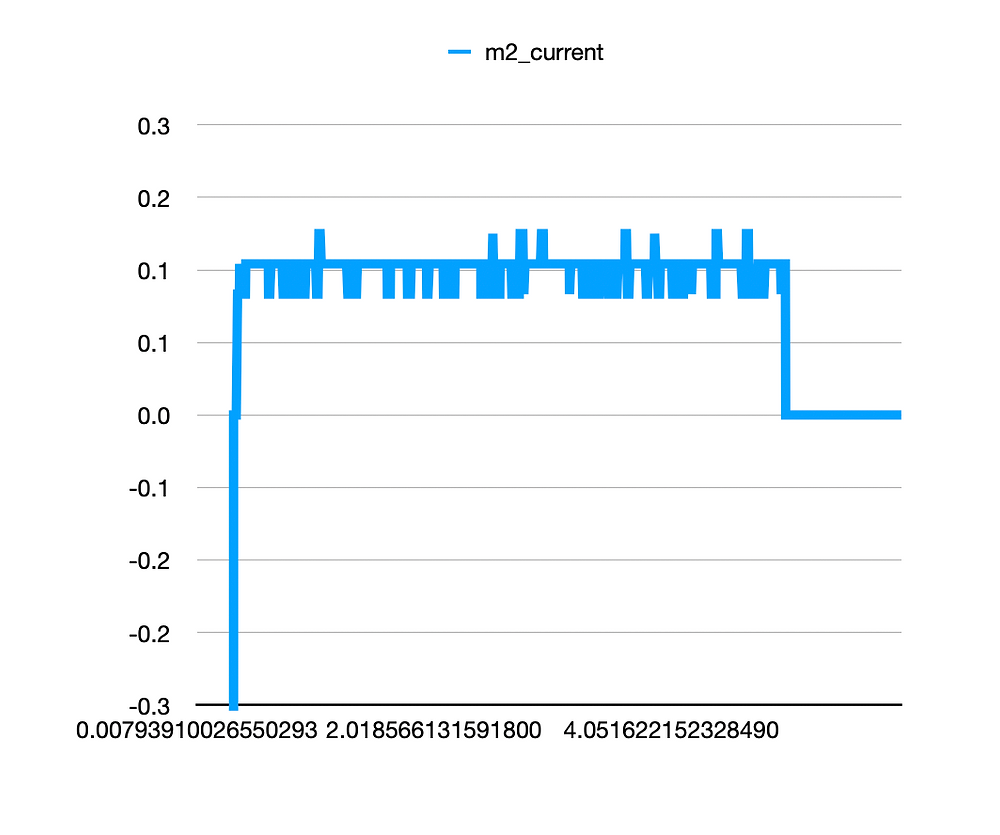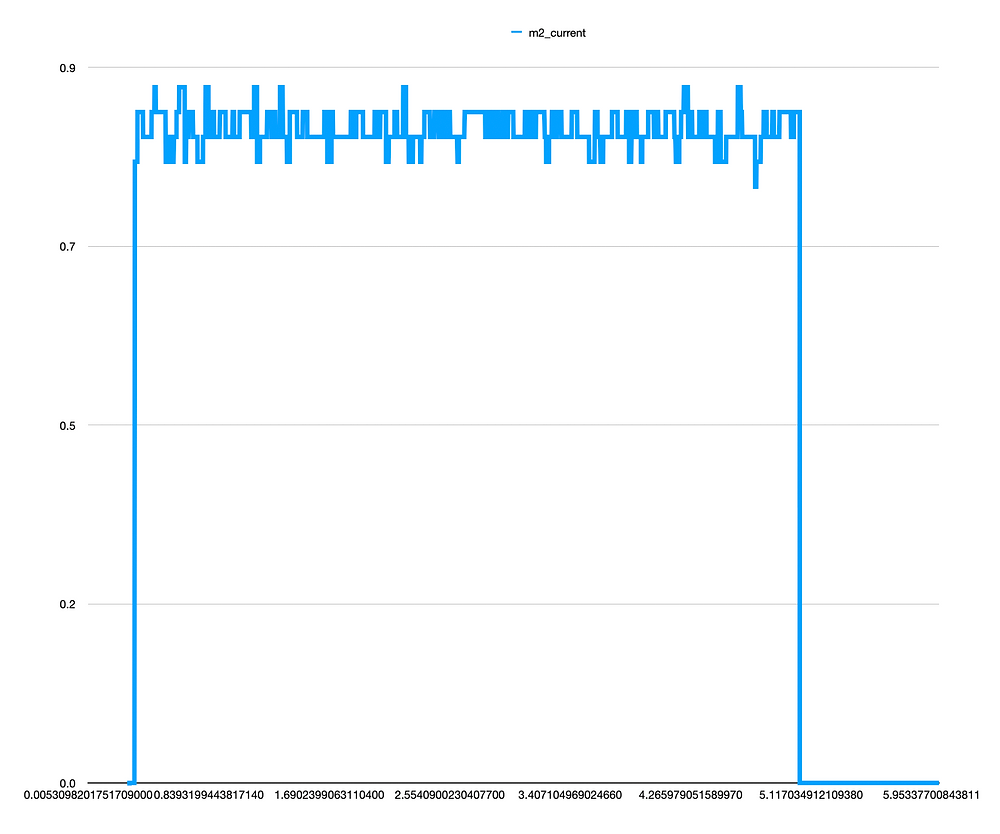Search
• aditya mehrotra.

# dyno updates: fixing the update rate of the system (RIO loop speed) [updates]

So we want to make the RIO run as fast as it possibly can right, we've estimated according to blog posts the RIO is capable of ~100Hz loop speed which should be enough for our purposes. That's a delay of ~0.01 (or half of what it is now, currently the system runs @ 50Hz).

```
Check out these links:
​Creating a Robot Program — FIRST Robotics Competition documentation (wpilib.org)
TimedRobot (WPILib API 2021.3.1)​

```

Both of them tell us a little about how exactly to update the loop speed. But because WPILIB has gotten better over the years we want to see how fast we can really make this loop go. This thread seems to suggest we can go up to even 200Hz which would be ideal. https://www.chiefdelphi.com/t/loop-time-of-0-02s-overrun-warning-message-question-about-robots-default-period-and-how-fast-should-the-roborio-loop-for-mp/340022/7

We're going to try 200Hz and see how that works. This is perfect because we want to be able to do PID control on the rio as opposed to on the motor controller which means we need to really up the loop-speed for that. The CAN should be OK even though there are a lot of devices on the bus (12x).

Here's a code block from the above information we're going to fix in our system to increase the loop speed:

```
public Robot() {
super(0.005); // Periodic methods will now be called every 30 ms.
}

```

The above method is the actual constructor of the class Robot() that we wrote before essentially we just stick this constructor after the networking instance but before the rest of the class methods.Ok well let's try it haha, had to confirm my java knowledge with my little brother who is a lot better at programming than I am ;)! Now we want to be able to check the logging speed after this update!So this above graph shows that the value of the actual logging period is around 0.01060199737 seconds which corresponds to a NT update rate of 94Hz. This is basically what we can expect since we programmed in ~ 100Hz that's not bad!

Now that we've fixed the loop speed and the NT update rate we can focus on getting the Kp Ki Kd values for tuning. We may not do this over NT as this will only slow the system down so we're just going to hardcode different values for current as well as position. We're going to command a current and log that current (Kp seems very high at the moment since we're getting wild oscillations in the controller, check out the graphs from last time to see). Let's just drop the Kp and see what happens for now (currently we have Kp Ki and Kd for the motor under test and the position hold motor as separate so we'll just keep it like that).So this Kp is clearly way too low. This is low by about a factor of 10 since the system saturates at 0.1 and we commanded 1.0 A to the motor. We're going to increase Kp and add Kd! Kp = 0.05 and Kd = 0.001!So Kd clearly needs more but Kp is looking ok? Let's try Kd = 0.005. Still looked like there were oscillations so we'll increase Kd more! Going to keep tuning until we reach a stable current controller.Now this above is with Kp = 0.01 and KD = 0.05, maybe increasing KD even more??? Dropping Kp a little? It's also possible we should tune the current controller with a completely locked rotor to get the best results? Right now it can oscillate since the other motor has some stiffness? Let's keep trying for a second or we'll up that KP to very high.

___ OK CHANGE OF PLANS BASED ON AN OBSERVATION ___

We probably could tune the current controller based on PID values right. Or we could relate the voltage to the sensed current by measuring it, here's graph of current based on a set voltage of 1.0V:The current is between 33-34 A of phase current when the voltage is set to 1.0! That means we can get an effective current controller by setting the voltage to the following:

```
V = 1.0 V, I = 33.5 A
C_set (V/A) = 1.0/33.5 V/A
Kt_eff = 1.0/33.5*Kt_motor

```

Let's say we want 1.0 A of current, we can set the voltage to 1.0/33.5 * 1.0 V, let's try this as an experiment.Ok this didn't work for the lower current, but honestly we will never be setting a current this low. Let's try 10.0A!!! That's about 0.1Nm!!!Now both of these graphs are about 10.0x lower than the desired current value? Let's see what happens if we multiply that Kt we calculated by 10.Ok so this was like commanding 100A with the old controller or 10.0A with the new controller. Still doesn't seem right, let's see what happens if we command 33.5A - maybe the curve is not linear!Yea this seems reasonable. OK so what we're going to do is command various voltages and measure the current, then we can curve-fit a curve as a voltage/current feed-forward controller for this motor. We're going to sweep from 0.0 to 5.0V for now!So if we look at the graphs above, we can see that the system starts out as a somewhat exponential curve and then goes linear, and then saturates at around 80A for some reason. It looks like there's an 80A current limit set at the output of the motor controller in this case!From this page: https://www.revrobotics.com/neo-brushless-motor-locked-rotor-testing/ the max current limit is around 80A so that may be the default in our case!!! From our torque sensor we got about 1.6 Nm max torque which translates to 16Nm out of the 10:1 gearbox! We're going to find out if this is enough (hopefully it is). Note that holding at the max current limit for 40s may cause motor failure like it shows here (w/ a locked rotor). According to out calculations we should see around 2Nm from 80A of motor current!!! So we're close but we have another 0.4Nm we're losing somewhere (but this measurement may be skewed by the fact that the rotor isn't locked). Let's try w/ a locked rotor. Even when holding the shaft the system peaked at around 1.6Nm!!!The other thing we noticed is that it takes the system when starting up (python) ~ 0.27 seconds to establish a connection with NT so the initial values don't really get counted! So let's send 0.0V for about 0.5s to get a better curve!So here's the final graph we obtained from the motor clearly there's an 80A current limit and we probably don't want to go more than that. We can use this essentially as a look-up table for current data to voltage data if we curve-fit this!

```
Important Saturation Data:
Lower Saturation: N/A
Upper Saturation: 80.0A @ > 8.0V

Important Precision Data:
Removed Data Below: 0.6V @ 0.6A
**data below this point is meaningless

```

So after we removed the saturations and all data that seemed meaningless, we end up with the following graph w/ the polynomial trend line labeled below.Below is the trend-line equation as generated by Numbers (hey it's superior to Excel + you know it).This equation is valid under locked rotor from 0.0 - 3.0V! But something tells me we'll have to actually tune a current controller because this equations very likely varies with the speed of the motor (it must). As the speed increases, the torque must decrease therefor this is actually a pretty useless curve-fit but interesting nonetheless. But at least we now have an idea of how to tune the current controller. PID current controller will have to be the way to go then, it might be worth only tuning the current controller to achieve the desired current as a RUNNING AVERAGE or something, we'll have to figure that out.

2 views0 comments

### Recent Posts

See All

#### chip updates: combining a kangaroo, a ship pulley, and a wild robot arm into a leg [updates]

© copyright 2019 | aditya mehrotra